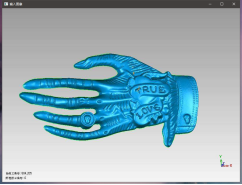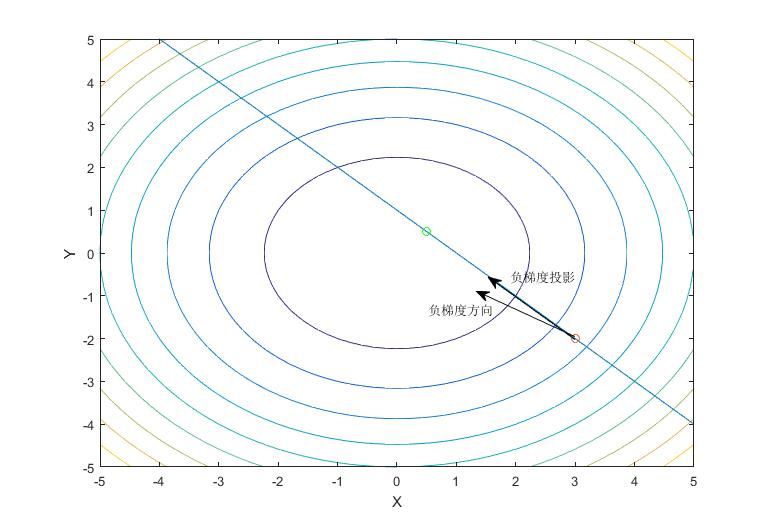• /* Sobel算子API实现求图像XY方向梯度 */ /************************************************************************/ int main() { //cv::Mat matRgb(h, w, CV_8UC3); //cv::Mat matGray(h, w, CV_8UC1); ...
OpenCV+VS 代码如下：
#include <cvInclude.h>
#include <iostream>
using namespace cv;

/************************************************************************/
/*                    Sobel算子API实现求图像X与Y方向梯度                    */
/************************************************************************/

int main()
{
//cv::Mat matRgb(h, w, CV_8UC3);
//cv::Mat matGray(h, w, CV_8UC1);
//cv::Mat matShort(h, w, CV_16S);

if (!inImg.data)
{
return -1;
}
imshow("输入图像",inImg);
int height = inImg.rows;//输入图像的高
int weight = inImg.cols;//输入图像的宽

//求X方向梯度
//第3个参数：int类型的ddepth，输出图像的深度
//第4个参数：int类型dx，x方向的差分阶数；
//第5个参数：int类型dy，y方向的差分阶数；
//第6个参数：int类型ksize，默认值3，表示Sobel核的大小；必须取1，3，5，7
//第7个参数：double类型scale，计算导数值时可选的缩放因子，默认为1
//第8个参数：double类型delta，表示在结果存入目标图(dst)之前可选的delta值，默认值0
//第9个参数：int类型的borderType，边界模式，默认为BORDER_DEFAULT
Sobel(inImg, grad_x, CV_16S, 1, 0, 3, 1, 1, BORDER_DEFAULT);
//必须取绝对值，否则输出全灰色。我猜是因为：梯度有方向

//求Y方向梯度
Sobel(inImg, grad_y, CV_16S, 0, 1, 3, 1, 1, BORDER_DEFAULT);

waitKey(0);
return 0;

}
输出结果：展开全文Sobel
• ## 梯度

千次阅读 2018-01-28 22:35:31
 1. 梯度
在微积分里面，对多元函数的参数求∂偏导数，把求得的各个参数的偏导数以向量的形式写出来，就是梯度。比如函数f(x,y), 分别对x,y求偏导数，求得的梯度向量就是(∂f/∂x, ∂f/∂y)T,简称grad f(x,y)或者▽f(x,y)。对于在点(x0,y0)的具体梯度向量就是(∂f/∂x0, ∂f/∂y0)T.或者▽f(x0,y0)，如果是3个参数的向量梯度，就是(∂f/∂x, ∂f/∂y，∂f/∂z)T,以此类推。
那么这个梯度向量求出来有什么意义呢？他的意义从几何意义上讲，就是函数变化增加最快的地方。具体来说，对于函数f(x,y),在点(x0,y0)，沿着梯度向量的方向就是(∂f/∂x0, ∂f/∂y0)T的方向是f(x,y)增加最快的地方。或者说，沿着梯度向量的方向，更加容易找到函数的最大值。反过来说，沿着梯度向量相反的方向，也就是 -(∂f/∂x0, ∂f/∂y0)T的方向，梯度减少最快，也就是更加容易找到函数的最小值。

2. 梯度下降与梯度上升
在机器学习算法中，在最小化损失函数时，可以通过梯度下降法来一步步的迭代求解，得到最小化的损失函数，和模型参数值。反过来，如果我们需要求解损失函数的最大值，这时就需要用梯度上升法来迭代了。
梯度下降法和梯度上升法是可以互相转化的。比如我们需要求解损失函数f(θ)的最小值，这时我们需要用梯度下降法来迭代求解。但是实际上，我们可以反过来求解损失函数 -f(θ)的最大值，这时梯度上升法就派上用场了。
下面来详细总结下梯度下降法。
3. 梯度下降法算法详解
3.1 梯度下降的直观解释
首先来看看梯度下降的一个直观的解释。比如我们在一座大山上的某处位置，由于我们不知道怎么下山，于是决定走一步算一步，也就是在每走到一个位置的时候，求解当前位置的梯度，沿着梯度的负方向，也就是当前最陡峭的位置向下走一步，然后继续求解当前位置梯度，向这一步所在位置沿着最陡峭最易下山的位置走一步。这样一步步的走下去，一直走到觉得我们已经到了山脚。当然这样走下去，有可能我们不能走到山脚，而是到了某一个局部的山峰低处。
从上面的解释可以看出，梯度下降不一定能够找到全局的最优解，有可能是一个局部最优解。当然，如果损失函数是凸函数，梯度下降法得到的解就一定是全局最优解。3.2 梯度下降的相关概念
在详细了解梯度下降的算法之前，我们先看看相关的一些概念。
1. 步长（Learning rate）：步长决定了在梯度下降迭代的过程中，每一步沿梯度负方向前进的长度。用上面下山的例子，步长就是在当前这一步所在位置沿着最陡峭最易下山的位置走的那一步的长度。
2.特征（feature）：指的是样本中输入部分，比如样本（x0,y0）,（x1,y1）,则样本特征为x，样本输出为y。
3. 假设函数（hypothesis function）：在监督学习中，为了拟合输入样本，而使用的假设函数，记为hθ(x)。比如对于样本（xi,yi）(i=1,2,...n),可以采用拟合函数如下： hθ(x) = θ0+θ1x。
4. 损失函数（loss function）：为了评估模型拟合的好坏，通常用损失函数来度量拟合的程度。损失函数极小化，意味着拟合程度最好，对应的模型参数即为最优参数。在线性回归中，损失函数通常为样本输出和假设函数的差取平方。比如对于样本（xi,yi）(i=1,2,...n),采用线性回归，损失函数为：

J(θ0,θ1)=∑i=1m(hθ(xi)−yi)2
J(θ0,θ1)=∑i=1m(hθ(xi)−yi)2
其中

xi
xi表示样本特征x的第i个元素，

yi
yi表示样本输出y的第i个元素，

hθ(xi)
hθ(xi)为假设函数。
3.3 梯度下降的详细算法
梯度下降法的算法可以有代数法和矩阵法（也称向量法）两种表示，如果对矩阵分析不熟悉，则代数法更加容易理解。不过矩阵法更加的简洁，且由于使用了矩阵，实现逻辑更加的一目了然。这里先介绍代数法，后介绍矩阵法。

3.3.1 梯度下降法的代数方式描述
1. 先决条件： 确认优化模型的假设函数和损失函数。
比如对于线性回归，假设函数表示为

hθ(x1,x2,...xn)=θ0+θ1x1+...+θnxn
hθ(x1,x2,...xn)=θ0+θ1x1+...+θnxn, 其中

θi
θi (i = 0,1,2... n)为模型参数，

xi
xi (i = 0,1,2... n)为每个样本的n个特征值。这个表示可以简化，我们增加一个特征

x0=1
x0=1 ，这样

hθ(x0,x1,...xn)=∑i=0nθixi
hθ(x0,x1,...xn)=∑i=0nθixi。
同样是线性回归，对应于上面的假设函数，损失函数为：

J(θ0,θ1...,θn)=12m∑i=0m(hθ(x0,x1,...xn)−yi)2
J(θ0,θ1...,θn)=12m∑i=0m(hθ(x0,x1,...xn)−yi)2

2. 算法相关参数初始化：主要是初始化

θ0,θ1...,θn
θ0,θ1...,θn,算法终止距离

ε
ε以及步长

α
α。在没有任何先验知识的时候，我喜欢将所有的

θ
θ初始化为0， 将步长初始化为1。在调优的时候再 优化。
3. 算法过程：
1）确定当前位置的损失函数的梯度，对于

θi
θi,其梯度表达式如下：

∂∂θiJ(θ0,θ1...,θn)
∂∂θiJ(θ0,θ1...,θn)
2）用步长乘以损失函数的梯度，得到当前位置下降的距离，即

α∂∂θiJ(θ0,θ1...,θn)
α∂∂θiJ(θ0,θ1...,θn)对应于前面登山例子中的某一步。
3）确定是否所有的

θi
θi,梯度下降的距离都小于

ε
ε，如果小于

ε
ε则算法终止，当前所有的

θi
θi(i=0,1,...n)即为最终结果。否则进入步骤4.
4）更新所有的

θ
θ，对于

θi
θi，其更新表达式如下。更新完毕后继续转入步骤1.

θi=θi−α∂∂θiJ(θ0,θ1...,θn)
θi=θi−α∂∂θiJ(θ0,θ1...,θn)
下面用线性回归的例子来具体描述梯度下降。假设我们的样本是

(x(0)1,x(0)2,...x(0)n,y0),(x(1)1,x(1)2,...x(1)n,y1),...(x(m)1,x(m)2,...x(m)n,yn)
(x1(0),x2(0),...xn(0),y0),(x1(1),x2(1),...xn(1),y1),...(x1(m),x2(m),...xn(m),yn),损失函数如前面先决条件所述：

J(θ0,θ1...,θn)=12m∑i=0m(hθ(x0,x1,...xn)−yi)2
J(θ0,θ1...,θn)=12m∑i=0m(hθ(x0,x1,...xn)−yi)2。
则在算法过程步骤1中对于

θi
θi 的偏导数计算如下：

∂∂θiJ(θ0,θ1...,θn)=1m∑j=0m(hθ(xj0,xj1,...xjn)−yj)xji
∂∂θiJ(θ0,θ1...,θn)=1m∑j=0m(hθ(x0j,x1j,...xnj)−yj)xij
由于样本中没有

x0
x0上式中令所有的

xj0
x0j为1.
步骤4中

θi
θi的更新表达式如下：

θi=θi−α1m∑j=0m(hθ(xj0,xj1,...xjn)−yj)xji
θi=θi−α1m∑j=0m(hθ(x0j,x1j,...xnj)−yj)xij
从这个例子可以看出当前点的梯度方向是由所有的样本决定的，加

1m
1m 是为了好理解。由于步长也为常数，他们的乘机也为常数，所以这里

α1m
α1m可以用一个常数表示。
在下面第4节会详细讲到的梯度下降法的变种，他们主要的区别就是对样本的采用方法不同。这里我们采用的是用所有样本。
3.3.2 梯度下降法的矩阵方式描述
这一部分主要讲解梯度下降法的矩阵方式表述，相对于3.3.1的代数法，要求有一定的矩阵分析的基础知识，尤其是矩阵求导的知识。
1. 先决条件： 和3.3.1类似， 需要确认优化模型的假设函数和损失函数。对于线性回归，假设函数

hθ(x1,x2,...xn)=θ0+θ1x1+...+θnxn
hθ(x1,x2,...xn)=θ0+θ1x1+...+θnxn的矩阵表达方式为：

hθ(x)=Xθ
hθ(x)=Xθ ，其中， 假设函数

hθ(X)
hθ(X)为mx1的向量,

θ
θ为nx1的向量，里面有n个代数法的模型参数。

X
X为mxn维的矩阵。m代表样本的个数，n代表样本的特征数。
损失函数的表达式为：

J(θ)=12(Xθ−Y)T(Xθ−Y)
J(θ)=12(Xθ−Y)T(Xθ−Y), 其中

Y
Y是样本的输出向量，维度为mx1.
2. 算法相关参数初始化:

θ
θ向量可以初始化为默认值，或者调优后的值。算法终止距离

ε
ε，步长

α
α和3.3.1比没有变化。
3. 算法过程：
1）确定当前位置的损失函数的梯度，对于

θ
θ向量,其梯度表达式如下：

∂∂θJ(θ)
∂∂θJ(θ)
2）用步长乘以损失函数的梯度，得到当前位置下降的距离，即

α∂∂θJ(θ)
α∂∂θJ(θ)对应于前面登山例子中的某一步。
3）确定

θ
θ向量里面的每个值,梯度下降的距离都小于

ε
ε，如果小于

ε
ε则算法终止，当前

θ
θ向量即为最终结果。否则进入步骤4.
4）更新

θ
θ向量，其更新表达式如下。更新完毕后继续转入步骤1.

θ=θ−α∂∂θJ(θ)
θ=θ−α∂∂θJ(θ)

还是用线性回归的例子来描述具体的算法过程。
损失函数对于

θ
θ向量的偏导数计算如下：

∂∂θJ(θ)=XT(Xθ−Y)
∂∂θJ(θ)=XT(Xθ−Y)
步骤4中

θ
θ向量的更新表达式如下：

θ=θ−αXT(Xθ−Y)
θ=θ−αXT(Xθ−Y)
对于3.3.1的代数法，可以看到矩阵法要简洁很多。这里面用到了矩阵求导链式法则，和两个矩阵求导的公式。
公式1：

∂∂X(XXT)=2X
∂∂X(XXT)=2X
公式2：

∂∂θ(Xθ)=XT
∂∂θ(Xθ)=XT
如果需要熟悉矩阵求导建议参考张贤达的《矩阵分析与应用》一书。

3.4 梯度下降的算法调优
在使用梯度下降时，需要进行调优。哪些地方需要调优呢？
1. 算法的步长选择。在前面的算法描述中，我提到取步长为1，但是实际上取值取决于数据样本，可以多取一些值，从大到小，分别运行算法，看看迭代效果，如果损失函数在变小，说明取值有效，否则要增大步长。前面说了。步长太大，会导致迭代过快，甚至有可能错过最优解。步长太小，迭代速度太慢，很长时间算法都不能结束。所以算法的步长需要多次运行后才能得到一个较为优的值。
2. 算法参数的初始值选择。 初始值不同，获得的最小值也有可能不同，因此梯度下降求得的只是局部最小值；当然如果损失函数是凸函数则一定是最优解。由于有局部最优解的风险，需要多次用不同初始值运行算法，关键损失函数的最小值，选择损失函数最小化的初值。
3.归一化。由于样本不同特征的取值范围不一样，可能导致迭代很慢，为了减少特征取值的影响，可以对特征数据归一化，也就是对于每个特征x，求出它的期望

x¯¯¯
x¯和标准差std(x)，然后转化为：

x−x¯¯¯std(x)
x−x¯std(x)
这样特征的新期望为0，新方差为1，迭代次数可以大大加快。
4. 梯度下降法大家族（BGD，SGD，MBGD）
批量梯度下降法，是梯度下降法最常用的形式，具体做法也就是在更新参数时使用所有的样本来进行更新，这个方法对应于前面3.3.1的线性回归的梯度下降算法，也就是说3.3.1的梯度下降算法就是批量梯度下降法。

θi=θi−α∑j=0m(hθ(xj0,xj1,...xjn)−yj)xji
θi=θi−α∑j=0m(hθ(x0j,x1j,...xnj)−yj)xij
由于我们有m个样本，这里求梯度的时候就用了所有m个样本的梯度数据。
随机梯度下降法，其实和批量梯度下降法原理类似，区别在与求梯度时没有用所有的m个样本的数据，而是仅仅选取一个样本j来求梯度。对应的更新公式是：

θi=θi−α(hθ(xj0,xj1,...xjn)−yj)xji
θi=θi−α(hθ(x0j,x1j,...xnj)−yj)xij
随机梯度下降法，和4.1的批量梯度下降法是两个极端，一个采用所有数据来梯度下降，一个用一个样本来梯度下降。自然各自的优缺点都非常突出。对于训练速度来说，随机梯度下降法由于每次仅仅采用一个样本来迭代，训练速度很快，而批量梯度下降法在样本量很大的时候，训练速度不能让人满意。对于准确度来说，随机梯度下降法用于仅仅用一个样本决定梯度方向，导致解很有可能不是最优。对于收敛速度来说，由于随机梯度下降法一次迭代一个样本，导致迭代方向变化很大，不能很快的收敛到局部最优解。
那么，有没有一个中庸的办法能够结合两种方法的优点呢？有！这就是4.3的小批量梯度下降法。
小批量梯度下降法是批量梯度下降法和随机梯度下降法的折衷，也就是对于m个样本，我们采用x个样子来迭代，1<x<m。一般可以取x=10，当然根据样本的数据，可以调整这个x的值。对应的更新公式是：

θi=θi−α∑j=tt+x−1(hθ(xj0,xj1,...xjn)−yj)xji
θi=θi−α∑j=tt+x−1(hθ(x0j,x1j,...xnj)−yj)xij
5. 梯度下降法和其他无约束优化算法的比较
在机器学习中的无约束优化算法，除了梯度下降以外，还有前面提到的最小二乘法，此外还有牛顿法和拟牛顿法。
梯度下降法和最小二乘法相比，梯度下降法需要选择步长，而最小二乘法不需要。梯度下降法是迭代求解，最小二乘法是计算解析解。如果样本量不算很大，且存在解析解，最小二乘法比起梯度下降法要有优势，计算速度很快。但是如果样本量很大，用最小二乘法由于需要求一个超级大的逆矩阵，这时就很难或者很慢才能求解解析解了，使用迭代的梯度下降法比较有优势。
梯度下降法和牛顿法/拟牛顿法相比，两者都是迭代求解，不过梯度下降法是梯度求解，而牛顿法/拟牛顿法是用二阶的海森矩阵的逆矩阵或伪逆矩阵求解。相对而言，使用牛顿法/拟牛顿法收敛更快。但是每次迭代的时间比梯度下降法长。
（欢迎转载，转载请注明出处。欢迎沟通交流： pinard.liu@ericsson.com）

展开全文• ## 【最优化】梯度投影法的几何意义

万次阅读 热门讨论 2018-12-09 15:36:09


【最优化】梯度投影法
梯度投影法理论投影矩阵梯度投影法
例子几何意义不足之处参考资料

梯度投影法理论
投影矩阵
我觉得这篇文章写的还行 https://www.cnblogs.com/bigmonkey/p/9897047.html
梯度投影法
例子

min

⁡

f

(

x

)

=

x

2

+

y

2

s

.

t

.

x

+

y

≥

1

显然当

x

=

y

=

0.5

x=y=0.5

时有最小值，且

∇

f

(

x

)

=

(

2

x

1

2

x

2

)

\nabla f(x)= \left( %左括号 \begin{array}{cc} %该矩阵一共3列，每一列都居中放置 2x_1 \\ %第一行元素 2x_2 \\ %第二行元素 \end{array} \right)

这里我们假设初始点

x

(

1

)

=

(

3

,

−

2

)

x^{(1)}=(3,-2)

，积极约束矩阵

A

=

(

1

1

)

,

b

=

(

1

)

A=(1 \ \ \ 1),b=(1)

几何意义
该例的等高面如图所示其中，绿点是最小值点，红点是初始点，两条黑线箭头分别是负梯度方向

−

∇

f

(

x

)

-\nabla f(x)

和负梯度的投影方向

d

(

1

)

=

−

P

∇

f

(

x

)

d^{(1)}=-P\nabla f(x)

。这里P是投影矩阵，且

P

=

I

−

A

T

(

A

A

T

)

−

1

A

=

(

0.5

−

0.5

−

0.5

0.5

)

P=I-A^T(AA^T)^{-1}A= \left( %左括号 \begin{array}{cc} %该矩阵一共3列，每一列都居中放置 0.5 &amp; -0.5 \\ %第一行元素 -0.5 &amp; 0.5 \\ %第二行元素 \end{array} \right)

而

−

∇

f

(

x

(

1

)

)

=

(

−

6

4

)

-\nabla f(x^{(1)})= \left( %左括号 \begin{array}{cc} %该矩阵一共3列，每一列都居中放置 -6 \\ %第一行元素 4 \\ %第二行元素 \end{array} \right)

因此

d

(

1

)

=

−

P

∇

f

(

x

)

=

(

−

5

5

)

d^{(1)}=-P\nabla f(x)= \left( %左括号 \begin{array}{cc} %该矩阵一共3列，每一列都居中放置 -5 \\ %第一行元素 5 \\ %第二行元素 \end{array} \right)

实际上，

d

(

1

)

d^{(1)}

就是约束矩阵

A

A

的零空间的基。换言之，如果

−

P

∇

f

(

x

)

=

0

-P\nabla f(x)=0

，则当前点在约束下不存在下降方向。

−

P

∇

f

(

x

)

=

∇

f

(

x

)

-P\nabla f(x)=\nabla f(x)

，则当前点在约束下的下降方向就是梯度，即

∇

f

(

x

)

\nabla f(x)

在

A

A

的零空间上。

−

P

∇

f

(

x

)

≠

0

-P\nabla f(x)\ne 0

，则其为当前点在约束下的下降方向。
那么，为什么是这样呢？ 因为

A

A

是积极约束的矩阵，

A

A

的每一行都是一个向量（行向量），而

A

A

的零空间里的向量与这些向量都正交，该投影矩阵又恰好是

A

A

的零空间投影矩阵，能够把任何向量投影到零空间中（在此题中就是把梯度投影到零空间中）。比如，在上面的例子中，

A

A

的一个行向量是

(

1

1

)

(1\ \ \ \ 1)

，与其正交的向量是

(

−

k

k

)

,

k

∈

R

\left( %左括号 \begin{array}{cc} %该矩阵一共3列，每一列都居中放置 -k \\ %第一行元素 k \\ %第二行元素 \end{array} \right) ,k\in R

也即下降可行方向。
不足之处
如果梯度方向在约束范围的内部，会减速。比如在此例中如果把约束改为

x

&gt;

−

1

x&gt;-1

，初始点为

x

(

1

)

=

(

−

1

,

2

)

x^{(1)}=(-1,2)

时，梯度方向只需一次迭代，而梯度投影则还需要进行进一步判断（待更新）
参考资料
上海交大最优化讲义 陈宝林 《最优化理论与算法》
展开全文最优化 数学
• ## pytorch梯度

千次阅读 2019-07-06 17:02:12
tensor梯度的相关性
0   import torch
1   x=torch.ones(1)
3   y=x*w
5   output：
6    (False, True, True)


wx+b的梯度
x = torch.tensor(1., requires_grad=True)

# Build a computational graph.
y = w * x + b    # y = 2 * x + 3


全连接网络的梯度计算
x=torch.randn(10,3)#input
y=torch.randn(10,2)#output
#建立一个全连接层
linear=nn.Linear(3,2)#初始的w,b都是随机的
print(linear.weight,linear.bias)
#构造损失含税和优化器
criterion=nn.MSELoss()
optimizer=torch.optim.SGD(linear.parameters(),lr=0.01)#指定优化器优化的是全连接层的参数
#前向传播
pred=linear(x)
#计算loss
loss=criterion(pred,y)
print(loss.item())
#计算反向传播，此时还未进行梯度下降
loss.backward()
#梯度下降
optimizer.step()
#参数更新之后，再一次进行预测
pred=linear(x)
loss=criterion(pred,y)
print(loss.item())#经过一次梯度下降之后的预测loss


展开全文• TensorFlow2 手把手教你避开梯度消失和梯度爆炸.
• 举一个最简单的函数 y=x2y = x^2y=x2，梯度为 g(x)=∂yx=2xg(x) = \frac{\partial{y}}{\partial{x}} = 2xg(x)=∂xy​=2x。设学习率为 lrlrlr，那么 更新一次梯度后为：x1=x0−lr∗g(x0)=x0−lr∗2x0=(1−2∗lr)...
• ## pytorch 梯度计算

千次阅读 2018-12-23 19:11:00
梯度计算 import torch from torch.autograd ...x = Variable(torch.ones(2), requires_grad=True) # y = (4 * x * x).norm() y = (4 * x * x + 10 * x).mean() y.backward() print('x的值：',x) print('x梯度...pytorch
• 因此，对于一元函数，即y=f(x)，其梯度的方向总是指向x轴正方向或反方向，而大小即该点的导数。 如果考虑z=f(x,y)描绘的是一座在点(x,y)的高度为f(x,y)的山。那么，某一点的梯度方向是在该点坡度最陡的方向，而梯度...优化 数学优化 最速下降法
• 例如对于函数f(x,y)，分别对x,y求偏导，求得的梯度向量就是 (∂f/∂x, ∂f/∂y)^T，简称 grad f(x,y)，或者▽f(x,y)。相对于的，在点（x_0,y_0）处的梯度就是 (∂f/∂x_0, ∂f/∂y_0)^T, 或者记做 ▽f(x_0,y_0)。 2...机器学习 深度学习
• ## 偏导数与梯度

千次阅读 2019-02-07 12:36:15
文章目录偏导数[^1]链式法则梯度[^2]梯度...f(x,y)=x2y+sin⁡(y)f(x,y) = x^2y+\sin (y)f(x,y)=x2y+sin(y) 函数fff可以解释为yyy为自变量而xxx为常数的函数： f(x,y)=fx(y)=x2y+sin⁡(y)f(x,y)=f_x(y)=x^2y+\sin ...
• ## 图像梯度

千次阅读 2020-10-23 08:19:06
图像梯度原理：简单来说就是求导 OpenCV提供了三种不同的梯度滤波器，或者说高通滤波器：Sobel，Scharr和...可以设定求导的方向（xorder或yorder）。还可以设定使用的卷积核的大小（ksize），如果ksize=-1，会使用3x3
• 详细来讲讲梯度下降算法的原理，感受数学和程序的魅力吧！！
• ## 梯度与切向量

千次阅读 2018-06-27 15:06:34
之前一直错误的认为了切向量就是梯度，由于最近在看拉格朗日的方法，正好...此时需要写成参数式x=x 并且 y=x^2，分别对x求导数得到(1, 2x)把(a,b)带入即可。求点(a,b)处的梯度。此时需要写成f(x,y)=x^2-y，分别对...
• ## 梯度概念

千次阅读 2018-09-16 13:05:08
梯度的方向就是函数f(x,y)在这点增长最快的方向，梯度的模为方向导数的最大值。 梯度的本意是一个向量（矢量），表示某一函数在该点处的方向导数沿着该方向取得最大值，即函数在该点处沿着该方向（此梯度的方向）...
• 利用梯度下降算法求解y=x^2的极值。 注意：此种方法，除了x的更新之外，还有一点需要注意，那就迭代停止的条件。可以设置一个阈值a，比较x更新前后的y的差的绝对值与阈值a的大小，即Δy与a的大小。当Δy＜=a时，...
• 梯度下降 概念 梯度是一个向量，表示函数在...程序：使用梯度下降求解方程 y=x2−2x+1y=x2−2x+1 的最小值。 观察学习率对梯度下降的影响。 import matplotlib as mpl import matplotlib.pyplot as plt mpl.rcParam...
• ## 梯度的几何含义

千次阅读 2019-12-29 18:08:40
给定函数 f(x,y)=−(cos2x+cos2y)2f(x, y) = -(cos2x + cos 2 y )^2f(x,y)=−(cos2x+cos2y)2，则 ∇f\nabla f∇f 可以描述为在底部平面上的矢量投影（图片来源于Wikipedia）。 根据图片所示，每个点的梯度是一个...
• ## 随机梯度下降与动量详解

千次阅读 多人点赞 2018-05-23 15:18:33
1. SGD公式理解 注：这一部分引用自知乎用户Qi Qi，原回答链接 随机梯度下降主要用来求解类似于...f(x) = \sum_{i=1}^nf_i(w,x_i,y_i) 普通梯度下降算法： wt+1=wt−ηt+1∇f(wt)=wt−ηt+1∑i=1n∇fi(wt,xi...
• 梯度下降法的收敛性证明 ...函数y =f(x)为凸函数： 则： x1 = x-s*∇时 f(x1) < f(x0) 或 f(x1)>f(x0) 一定成立。 ∇指梯度，s指步长。 举例 如 y = x2 y = x2 是凸函数 当 x1 = x-s*∇时...
• ## pytorch-梯度

千次阅读 2020-04-21 02:48:57
import torch x = torch.ones(2, 2, requires_grad=True) print(x) ...y = x + 2 print(y) print(y.grad_fn) z = y * y * 3 out = z.mean() print(z, out) out.backward() # 等价于 out.backward(torch....java python 深度学习 神经网络 pytorch
• def createTrainingInstances(self, images): start = time.time() hog = cv2.HOGDescriptor() instances = [] for img, label in images: img = read_color_image(img) #函数未知 im...
• Ix(x,y)=I(x+1,y) - I(x-1,y), Iy(x,y) =I(x,y+1) - I(x,y-1), M(x,y) = sqrt(Ix(x,y)*Ix(x,y) +Iy(x,y)*Iy(x,y) ), Theta(x,y) = atan2(Iy(x,y), Ix(x,y)) 本文在编程实现这个简单的功能的同时，向没有SSE编程经验...c++代码 sse编程
• ## 梯度与梯度下降法

千次阅读 2018-05-26 22:07:45
• 一、问题描述 实现线性回归的梯度下降算法，解决糖尿病预测问题，输出mse和的值 ...X, y = load_diabetes(return_X_y = True) #获取数据 2、对数据进行训练，标准化处理 y = y.reshape((442, 1))...算法 数据挖掘 机器学习
• gradient descent函数是f(x)=x**4-3*x**3+2 python写法f(x)=x^4-3*(x^3)+2 C++ 写法导数是 f’(x)=4*x**3-9*x**2x从-50到50的图像 x从-5到5的图像 import numpy as np import matplotlib.pyplot as plt x=np.arang
• 1 分类 牛顿法(Newton‘s method) ...若函数f(x,y,z)在点P(x,y,z)处沿方向l(方向角为α、β、γ)，方向导数： 证明 2.2 梯度 2.2.1 定义 向量G称为函数f(P)在点P处的梯度(grad...优化算法 神经网络
• 本文总结了牛顿法, 拟牛顿法,梯度下降和随机梯度下降的概念和计算公式.牛顿法首先考虑一维问题. 对于一个一维的函数,如果这个函数连续可微, 并且导数可以计算, 那么计算这个函数的最优解可以看成求解方程f′(x)=0f...
• ## opencv 梯度方向

千次阅读 2019-08-05 13:26:46...